## Sunday, 21 July 2013

### The On-Board Sensor Interface & Interfacing External Sensors

The Induino R3 Board has an on-board sensor interface. The Sensor interface is to the Right of the microcontroller above the TSOP. If you look at it, it has pins in the following order
+5 - GND - A2  - A2 - +5 - GND

Connecting Other Variable Resistors - Using An External Resistor

Now to connect any other variable resistor type sensor in the voltage divider configuration, place one leg of the fixed resistor in GND and another leg in the A2 pin next to ground. Then place the variable resistor sensor between the next A2 Pin and +5V pin next to it.  That's it you are set to go. Look at this image of how we have connected a Force Sensitive Resistor. The programming for this would be the same as that of the LDR.

Interfacing a LM 35 Temperature Sensor

The LM35 is a temperature sensor that output a 10 millivolt / per degree C output. (Read the first page of the datasheet here). Here's a pin diagram for the LM35. The Flat side is facing up in the image.

Now Place the LM35 on the Sensor Interface Pins as shown in the image below.Bend the Gnd Pin (3rd Pin) a Little As Shown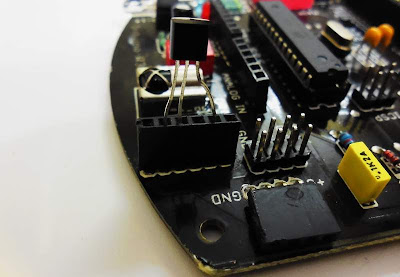Place the LM35 as Shown. This is the Flat Side of the LM35 Facing You. Note that we have skipped 1 pin in the sensor interface and plugged the LM35The Curved Side View of LM35 Placement.

Let's get to the programming now. The LM35 gives a linear output of 10 miilivolt per degree Celsius. It can measure temperature in the range of -55 to 150 degrees.  Our Analog Input Resolution is 10 bits. So we will be getting 0 to 1023 values for 0 to 5 volts.
0 to 1023 => 0 to 5 Volts

Therefore 1 Volt => 204.6  => 204 (this is the numerical value that represents 1 volt)
1 Volts => 1000 miiliVolt, So lets rewrite

1000 millivolt => 204
1 degree Celsius => 10 millivolts =>2.04 or 2 (So the numerical value 2 corresponds to 1 degree C )

So every increase in the numerical value signifies an increase in temperature by 0.5 degree. We shall  need to use this derivation in our calculation.

Here's our Program

``````/* Induino R3 User Guide - Program 6.0 - Simple LM 35 Temperature Sensor Interfacing */

float temp_val = 0; // variable to store the temperature value
void setup()-
{
Serial.begin(9600); // Initialise Serial Communication
}

void loop()
{
int sensor_val = analogRead(2); // Read the raw sensor value and store it in the variable
temp_val = sensor_val * 0.5; // The calculated value
Serial.print("Current Temperature : ");
Serial.println(temp_value); // print the temperature value to serial monitor
delay(1000); // a delay - do not remove this delay (you can change it) as otherwise the serial monitor will get flooded with data and might crash
}
``````

Increasing Precision

If you notice in the above program, our temperature measurement has a precision in the range of 0.5 degrees. What do we do if we require a  finer precision, say  0.1 degree? How do we get it?

Well if you noticed, the temperature range of LM35 is 150 degrees maximum  which corresponds to 1.5 volts, the LM35 will never output a value beyond 1.5 volts.  Now what if we can set the higher limit for our voltage value as 1.5 volts instead of 5 Volts? We would get an increased precision.

Analog Reference Voltage

Our ATmega 328 uses something called an Analog Reference Voltage. This is the voltage that is used as higher reference limit when working with Analog Inputs. By default this is the same as the operating voltage (5 Volts) however this can be over-ridden. You can set an Analog Reference Voltage other than the operating voltage in the following ways

Using the AREF Pin (below the GND pin below 13th Pin) - Connect an input producing any voltage but not exceeding the operating voltage. When an external voltage is connected to this pin, that voltage will be considered as the reference voltage.  You can try by connecting a wire from the 3.3 Volts pin to the AREF Pin.  Now,

3.3 Volts => 1023 Values
3300 Millivolts => 1023 Values
1 Degree => 10 Millivolts => 1023/330 Values => 3.1 or 3 Values
or 1 Value will correspond to 1/3 => 0.33 degrees

You can see that our precision has increased. Here's the program with calculation for 3.3V reference voltage

``````/* Induino R3 User Guide - Program 6.1 - LM 35 Temperature Sensor with Extenral Analog Reference Voltage of 3.3 volts*/

float temp_val = 0; // variable to store the temperature value

void setup()-
{
Serial.begin(9600); // Initialise Serial Communication
}

void loop()
{
int sensor_val = analogRead(2); // Read the raw sensor value and store it in the variable
temp_val = sensor_val * 0.33; // The calculated value
Serial.print("Current Temperature : ");
Serial.println(temp_value); // print the temperature value to serial monitor
delay(1000); // a delay - do not remove this delay (you can change it) as otherwise the serial monitor will get flooded with data and might crash
}
``````

Using the Internal Reference - The ATmega328 has the option to set the Analog Reference to Internal 1.1 volts. This can be done in the program. This would give us more precision with the LM35

0 to 1.1 volts => 0 - 1023 Values
1100 millivolts => 1023
1 Degree => 10 millivolts => 1023 / 110 => 9.3 or 9
or 1 Value will correspond to 1/9 => 0.11 degree

Now thats more precise than what we started with.

analogReference(INTERNAL) => This function sets the analog reference voltage to the internal 1.1 Volts

Heres the program for that

``````/* Induino R3 User Guide - Program 6.2 - LM 35 Temperature Sensor with Internal Analog Reference Voltage of 3.3 volts*/

float temp_val = 0; // variable to store the temperature value

void setup()
{
Serial.begin(9600); // Initialise Serial Communication
analogReference(INTERNAL); // Setup the Reference Voltage as Internal
}

void loop()
{
int sensor_val = analogRead(2); // Read the raw sensor value and store it in the variable
temp_val = sensor_val * 0.11; // The calculated value
Serial.print("Current Temperature : ");
Serial.println(temp_value); // print the temperature value to serial monitor
delay(1000); // a delay - do not remove this delay (you can change it) as otherwise the serial monitor will get flooded with data and might crash
}``````

Interfacing the HC-SR04 Ultrasonic Sensor

The HC-SR04 is a low cost ultrasonic sensor built along the lines of a PING Sensor.  When triggered, It sends out an ultrasonic sound wave through the ultrasonic transmitter and starts a timer,   The sound wave travels in a straigh line till it hits an obsctacle that reflects it back.  The reflected sound wave is received by the ultrasonic receiver. The timer is stopped once the signal is received by the receiver. The sensor, then gives a signal on the output pin. The duration of the signal on the output pin will correspond to the time taken by the ultrasonic sound wave to travel to and from the sensor. We can read this duration and calculate the distance of the obstacle

Programming the HC-SR04

We are going to use only 1 pin of the microcontroller for both Trig / Echo of the HC-SR04. So far, we saw that we used the pinMode() function in the setup() function. However, we can use the same pinMode() function in the loop function and change a pin from Input to Output at run-time.  The Sensor Interface on the Induino R3 Board uses the Pin A2  (or Digital 16 ) The HC-SR04 outputs a digital signal and so we will use the digital pin number in our program. Place the HC-SR04 as in the image below

Finally we need a new function

pulseIn(pin number, signal type) => the PulseIn() function returns the duration for which the given pin is either HIGH or LOW(HIGH or LOW can be the signal type parameters passed to the function) The duration is returned in microseconds.

Here's the program (this is the same as the PING Sensor program under examples)

``````/* Induino R3 User Guide - Program 6.3 - HC-SR04 Ultrasonic Sensor Interface*/

int pingPin = 16; // A2 - Connected to both Trig & ECHO Pins of the Sensor

void setup() {
// initialize serial communication:
Serial.begin(9600);
}

void loop()
{
// establish variables for duration of the ping,
// and the distance result in inches and centimeters:
long duration, inches, cm;

// The PING))) is triggered by a HIGH pulse of 2 or more microseconds.
// Give a short LOW pulse beforehand to ensure a clean HIGH pulse:
pinMode(pingPin, OUTPUT);
digitalWrite(pingPin, LOW);
delayMicroseconds(2);
digitalWrite(pingPin, HIGH); // Sending a High Pulse on the Trig Pin of the Sensor
delayMicroseconds(5);
digitalWrite(pingPin, LOW);

// The same pin is used to read the signal from the PING))): a HIGH
// pulse whose duration is the time (in microseconds) from the sending
// of the ping to the reception of its echo off of an object.
pinMode(pingPin, INPUT);
duration = pulseIn(pingPin, HIGH);

// convert the time into a distance
inches = microsecondsToInches(duration);
cm = microsecondsToCentimeters(duration);

Serial.print(inches);
Serial.print("in, ");
Serial.print(cm);
Serial.print("cm");
Serial.println();

delay(100);
}

long microsecondsToInches(long microseconds)
{
// According to Parallax's datasheet for the PING))), there are
// 73.746 microseconds per inch (i.e. sound travels at 1130 feet per
// second).  This gives the distance travelled by the ping, outbound
// and return, so we divide by 2 to get the distance of the obstacle.
// See: http://www.parallax.com/dl/docs/prod/acc/28015-PING-v1.3.pdf
return microseconds / 74 / 2;
}

long microsecondsToCentimeters(long microseconds)
{
// The speed of sound is 340 m/s or 29 microseconds per centimeter.
// The ping travels out and back, so to find the distance of the
// object we take half of the distance travelled.
return microseconds / 29 / 2;
}``````

Thats It For This Part! Enjoy... and feel free to drop us an email with questions you might have -> info@simplelabs.co.in

Visit www.simplelabs.co.in for more interesting products

Back to List of Contents

1.lm35 gives a analoge output . You connected to A2 .

The HC-SR04 is a digital output . You connected to A2 ! How is that ?
Also which physical pin is on the atmel chip? confused. Thanks

1.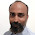Read the blog from the start :)

2.Ok I found that pin 25 of the AT328 = PC2 (ADC2/PCINT10) =A2 . So digitally your using PC2 .
Analog it is ADC2 . Am I correct? both A2

3.4.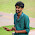I am connecting Hall effect current sensor ACS 712 into Induino R3 and interfacing with Matlab 2014 but i am unable to view that input in matlab. Any idea please...??

5.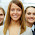This blog is so nice to me. I will keep on coming here again and again. Visit my link as well.

6.This comment has been removed by the author.

7.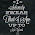if I want to display the sensor value(distance or temperature) in both serial monitor and 16*2 LCD display, what is the change I have to do in the above programs??

1.sorry i found about that in another blog, updated on another date,

2.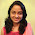This comment has been removed by the author.

8.This is really very nice idea. Thank you for sharing with us. Compression load cell has two types like CMP of monolith Construction, CMP-SP. Each has made with high nickel alloy steel. Some of the applications compression load cell used in object weighing, production lines, test systems and Torque supports.

9.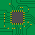Hi! I have a Temperature and Humidity sensor. Is it possible to know the Humidity with a program? If yes, please provide the program.

Thank you